# Parallel Closure Scheme

There are a number of other terms that appear in the so-called Braginskii equations, described in Section 2.6, that are subject to modification in a long mean-free-path plasma. .

Consider the thermal force density [see Equation (2.36)]: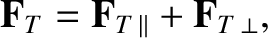(2.305)

where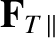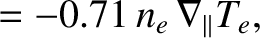(2.306)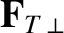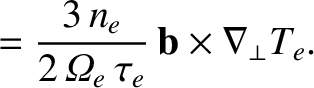(2.307)

Both the parallel and the perpendicular components of this force density are a consequence of the fact that the friction force density is inversely proportional to the temperatures of electrons that are scattered by ions [6,18].

Let us first examine the parallel component of the thermal force density. Suppose that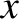measures distance along a magnetic field-line. We can write [6,18]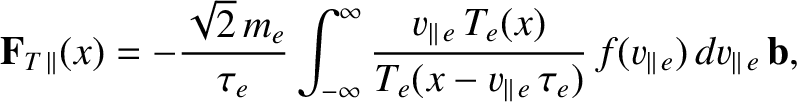(2.308)

where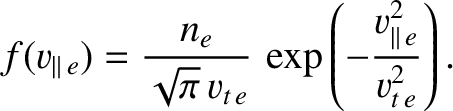(2.309)

Here, we are making use of the fact thatis sum of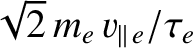(the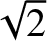factor is to get agreement with the collisional result), taken over all electrons moving parallel to the field-line, and weighted by the inverse of the electron temperature at the last scattering position. Thus, electrons with parallel velocity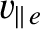that are scattered by ions at positionwere last scattered at position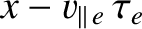, and are, therefore, characterized by the electron temperature at the latter position. Suppose that a tearing mode gives rise to a perturbation to the electron temperature along the field-line of the form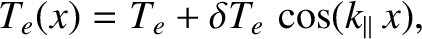(2.310)

where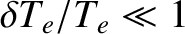. It follows that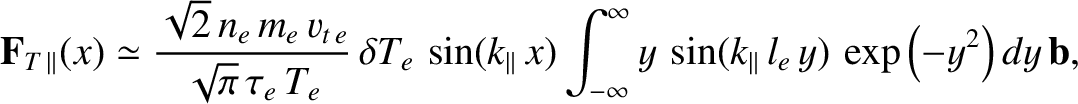(2.311)

which reduces to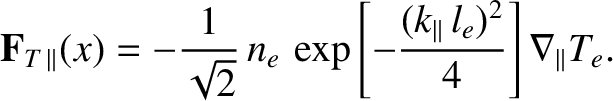(2.312)

Here, use has been made of the result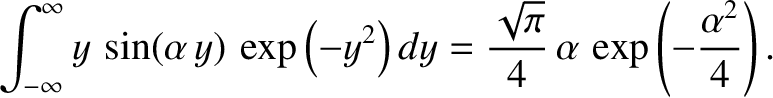(2.313)

It can be seen that, in the short mean-free-path limit,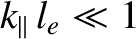, Equation (2.312) is identical to Equation (2.306). However, in the long mean-free-path limit,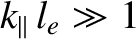, the parallel thermal force density is attenuated by a factor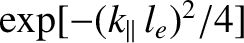. As is clear from Table 2.1, unless the parallel wavelength of the tearing mode exceeds a few kilometers (i.e., the electron mean-free-path), this attenuation is very strong in a tokamak fusion reactor. Now, tearing modes are global instabilities of tokamak plasmas, so they have wavelengths that do not differ greatly from the dimensions of the plasma, which is a few meters. Thus, we conclude that there is no parallel component of the thermal force density in a tokamak fusion reactor. To be slightly more exact,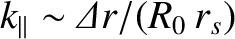for a tearing mode in a tokamak plasma, where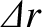measures radial distance from the rational surface, whose minor radius is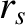, at which it resonates with the equilibrium magnetic field. (See Section 1.11.) Thus, the attenuation of the parallel thermal force is strong unless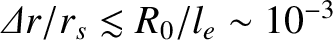.

Let us now consider the perpendicular component of the thermal force density. The appropriate calculation is analogous to that we just performed, except that electrons that move perpendicular to magnetic field-lines, and are scattered by ions at a given point in the plasma, can only have originated from points that are, at most, a few electron gyro-radii away from the given point. Hence, the appropriate attenuation factor is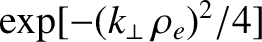. As is clear from Table 2.1, unless the perpendicular wavelength of the tearing mode falls below about 0.1 mm (i.e., the electron gyro-radius), there is no attenuation of the perpendicular thermal force density in a tokamak fusion reactor. Thus, we conclude that Equation (2.307) remains valid in such a reactor.

There is also a thermal component of the electron heat flux, which is specified in Equation (2.47). Similar arguments to those that we just made reveal that the parallel component of this heat flux is strongly attenuated in a tokamak fusion reactor, due to long mean-free-path effects, whereas the perpendicular component is unaffected.

Consider the diffusive parallel heat flux associated with the electron temperature perturbation (2.310). In the short mean-free-path limit, we have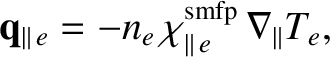(2.314)

where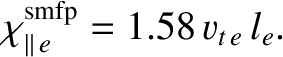(2.315)

[See Equations (2.44) and (2.48).] On the other hand, in the long mean-free-path (i.e., collisionless) limit [26,27]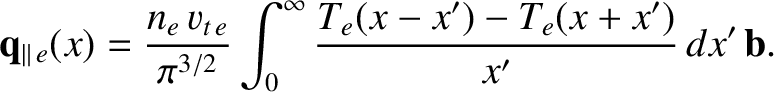(2.316)

It follows from Equation (2.310) that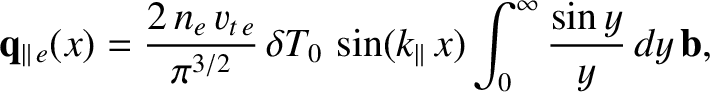(2.317)

which yields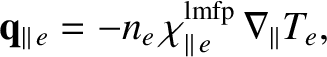(2.318)

where the long mean-free-path thermal conductivity takes the form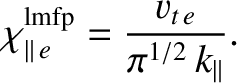(2.319)

Here, use has been made of the result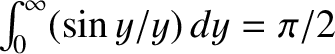. It can be seen, from a comparison between Equations (2.315) and (2.319), that the short mean-free-path parallel electron thermal diffusivity exceeds the collisionless diffusivity when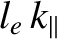exceeds about unity. However, this is impossible, because the collisionless diffusivity corresponds to the free flow of electrons along magnetic field-lines, completely unimpeded by collisions, and therefore sets an upper limit on the diffusivity. (Of course, in this limit, the parallel heat flow is not diffusive at all; instead, it is convective.) Hence, we conclude that in a tokamak fusion reactor, which is characterized by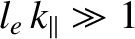, the appropriate expression for the parallel electron thermal “diffusivity” is given by Equation (2.319). Analogous arguments reveal that the ion parallel thermal “diffusivity” in a tokamak fusion reactor takes the form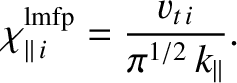(2.320)